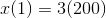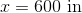7th Grade Math : Number Sense

Example Questions

Example Question #1 : Number Sense

A sweater was originally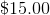, but the department store is running aoff sale. What is the sale price of the sweater?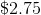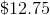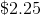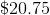Explanation:

In order to solve this problem, we need to calculateof. The key word "of" is indicative of multiplication; however, we need to start by converting the percentage into a decimal because you cannot multiply a number by a percent.

We can convert a percent into a decimal by moving the decimal two places to the left: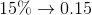Next, we can multiply: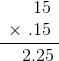Remember, we are takingoff the original price, which means we need to subtract.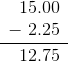Example Question #2 : 7th Grade Math

The rectangle provided is a scaled drawing of a rectangular yard. Given the scale of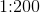, what is the actual length of the yard?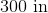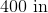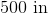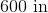Explanation:

This question is asking us to solve for the actual size of the length of the rectangle, therefore, we first need to recall which side is considered to be the length of the rectangle.In this example, the length of the rectangle is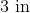. We can set up a proportion to solve for the actual length of the rectangle,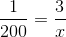Next, we cross multiply and solve for